Next: The Dynamic of the Up: The Mandelbrot set Previous: The Mandelbrot set

### Some properties of the Mandelbrot set

This section summons some properties of the Mandelbrot set first without proof, then some statements are proved.

Theorem 3 (Symmetry)   The Mandelbrot set is symmetric with respect to the real axis. This means, if a complex number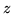belongs to the mandelbrot set then this is also true for the conjigate complex number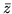. (You can see this symmetry in Figure 3)

Theorem 4 (Boundary)   The Mandelbrot set is bounded. You can easily proove, thet the set must lie in the interior of the circle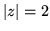. (Also see Figure 3)

Theorem 5 (Itself-Similarity)   The Mandelbrot set is itself similar in a non exact sense.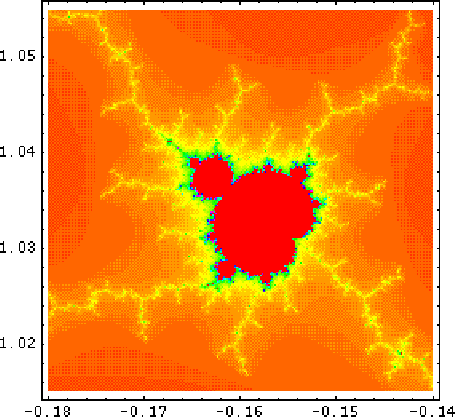Theorem 6 (Real axis)   The intersection of the Mandelbrot set with the real axis is the real interval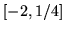Theorem 7 (connected set)   The mandelbrot set is a connected set. This result seems to contradict every picture of the set, where you think that the set consists of separated insuli.(See Figure 5)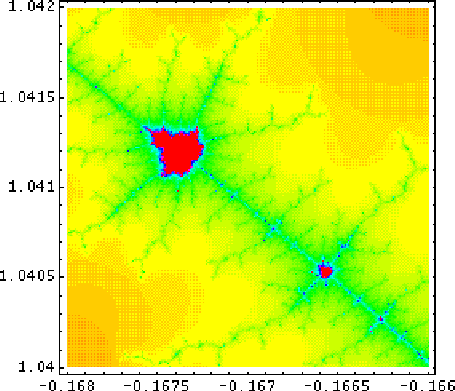Theorem 8   Fractal dimension of the border The border of the Mandelbrot set is a fractal structure with not yet known fractal dimension.Next: The Dynamic of the Up: The Mandelbrot set Previous: The Mandelbrot set
Tino Kluge
2000-12-05